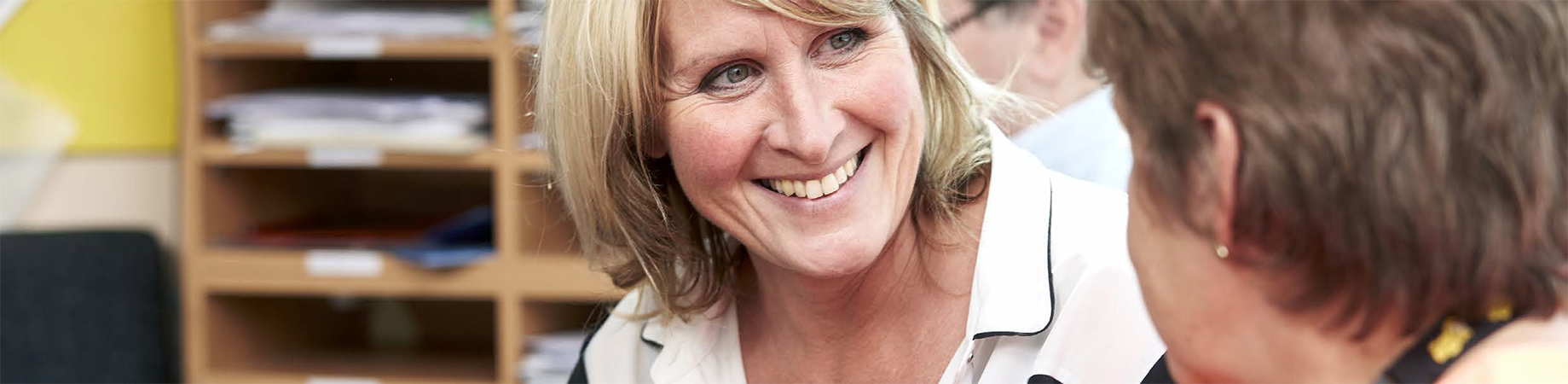# Mathematics (GCSE)

GCSE Mathematics

Name of Course
GCSE Mathematics A

Qualification
Edexcel GCSE Maths (9-1) 1MA1

Outline of Course
The Course is now a 3 year course, starting in Year 9, with examinations in the summer of Year 11. There are now 3 one and a half hour exam papers, Paper 1 is a non- calculator paper and papers 2 and 3 are calculator papers.
The new GCSE grades are from 1 to 5 in the Foundation course, a grade 4 being equivalent to a Grade C in the old exam.
Students may purchase revision guides and workbooks from school.

How it’s taught
Individual , paired work and group work activities.
Maths games, online activities and homework, using the mymaths website.

Topic Areas eg.

• Number
• Algebra
• Ratio & Proportion
• Geometry & Measures
• Probability and Statistics

Homework